12 篇文章 0 订阅

# MST（ minimum spanning tree）性质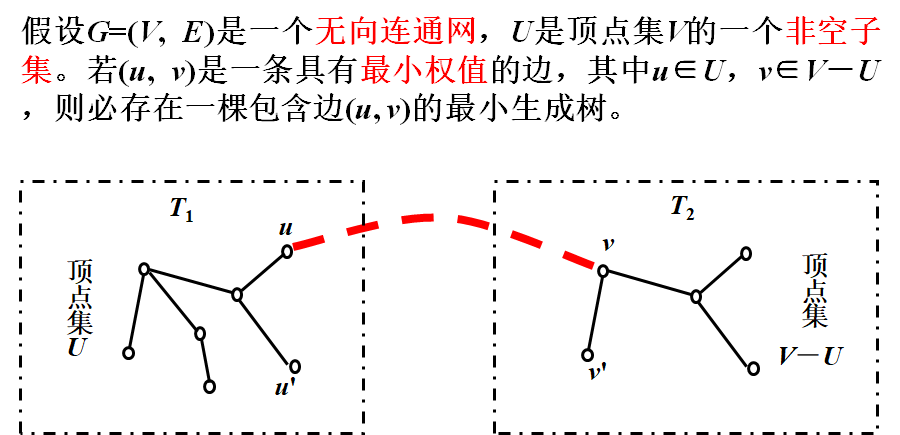# MST性质的应用

• Prime法：加点法
• Kruskal方法：加边法

## 1.普里姆（Prim）算法

T=(U, TE)是G的最小生成树，
T的初始状态为U={u0}（u0∈V），TE={ }，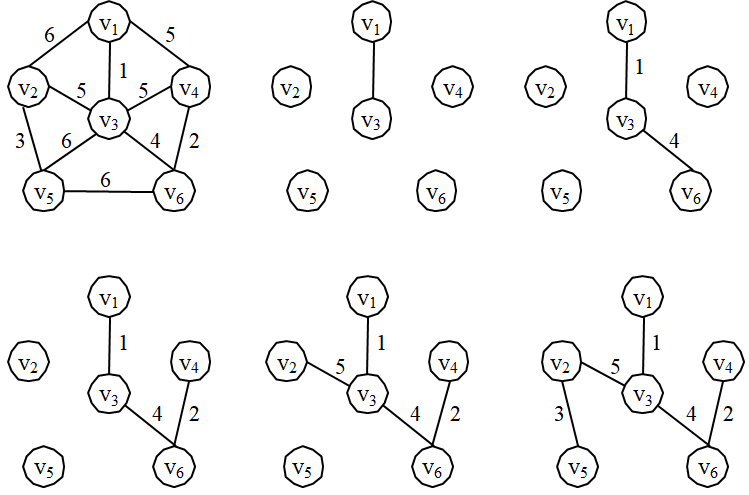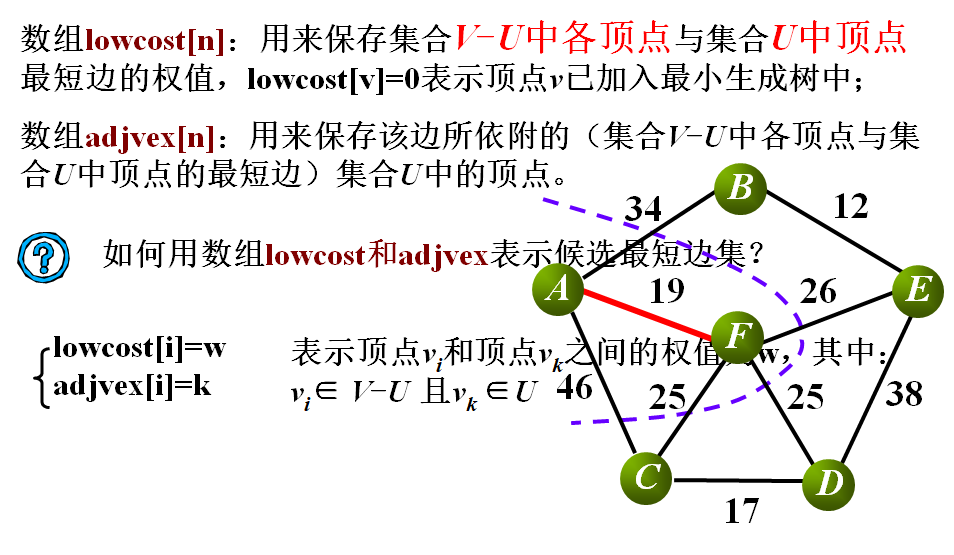## Prim算法——伪代码

2. 输出顶点u0，将顶点u0加入集合U中；
3. 重复执行下列操作n-1次
3.1 在lowcost中选取最短边(lowcost[k])，取对应的顶点序号k；
3.2 输出顶点k和对应的权值；
3.3 将顶点k加入集合U中（lowcost[k]=0）；
Void prime(MGraph G){
for(int i=1;i<G.vertexNu;i++){
}
lowcost=0;
for(i=1;i<G.vertexNum;i+++){
k=MinEdge(lowcost,G.vertexNum)
lowcost[k]=0
for(j=1;j<G.vertexNum;j++)
if((G.arc[k][j]<lowcost[j]){
lowcost[j]=G.arc[k][j];
arcvex[j]=k;
}
}
}


O（n2）

## 2.克鲁斯卡尔（Kruskal）算法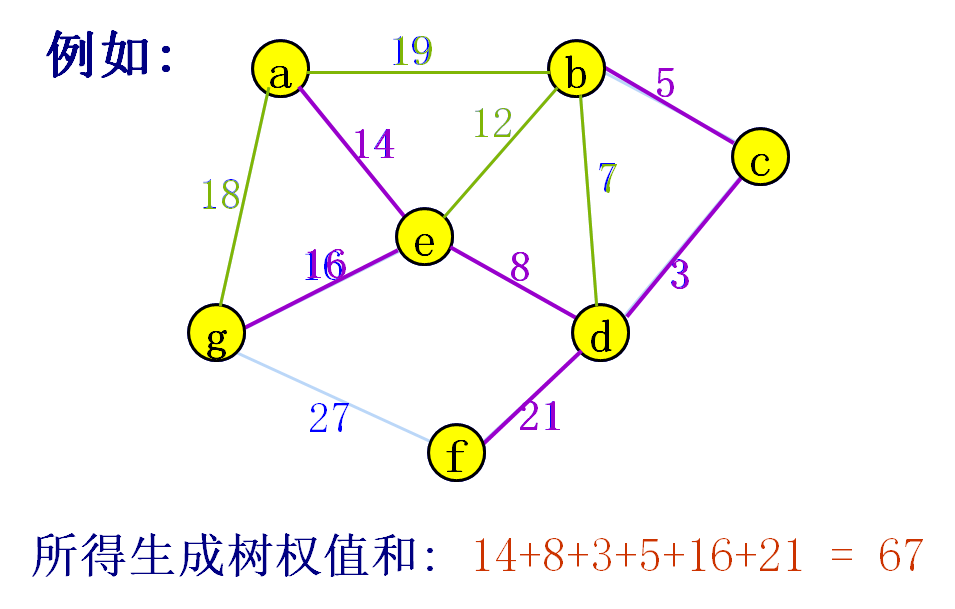### Kruskal算法实现中的三个关键问题

1. 图的存储结构
采用边集数组存储图。
2. 如何判断一条边所依附的两个顶点在同一个连通分两中(并查集)
定义Parent[i]数组。数组分量的值表示顶点i的双亲节点（初值为-1；）
当一条边（u,v）的两个顶点的根结不同时，这两个结点属于不同的连通分量（利用parent 数组查找一棵树的根节点。当一个结点n的parent==-1，树的根节点即为n）
3. 如何将一条边所依附的两个顶点合并到同一个连通分量中
要进行联通分量的合并 ，其中一个顶点所在的树的根节点为vex1，另一个顶点所在的树的根节点为vex2，则：parent[vex2]=vex1；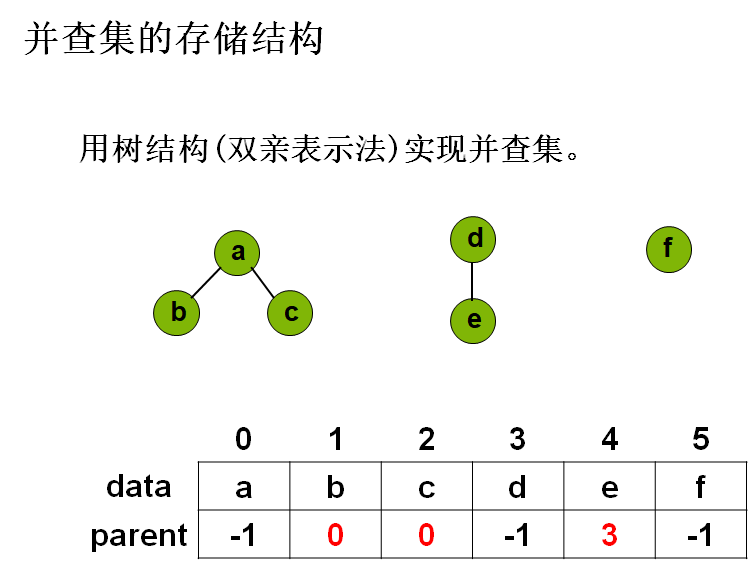int main(){
int arcNum, int vertexNum;
EdgeNode *edge;
int *parent;

cout<<"please input the number of vertexNum:"; cin>>vertexNum;
cout<<"please input the number of edges:";	cin>>arcNum;
edge=new EdgeNode[arcNum];	parent=new int[vertexNum];
for(int i=0;i<arcNum;i++)	{
cin>>edge[i].from>>edge[i].to>>edge[i].weight;
}
sort(edges, G); //对边集数组进行堆排序，时间复杂性为O(eloge)
for (i=0;i<vertexNum;i++)
parent[i]=-1;  //每个节点分属于不同的集合

int k=0,begin,end,count=0;
cout<<"next is the MST :"<<endl;
for (k=0;k<arcNum;k++)	{
begin=edge[k].from;	end=edge[k].to;
int m,n;
m=Find(parent,begin);	n=Find(parent,end);
if(m!=n)	{
cout<<begin<<","<<end<<","<<edge[k].weight<<endl;
parent[n]=m;
count++;
if(count==vertexNum-1)	break;
}
}
return 0;
}

int Find(int *parent, int node)
{
int f;
f=node;
while(parent[f]>-1)
f=parent[f];
return f;
}


### Kruskal算法的时间复杂性分析

12-12336001-15187
02-171万+
09-081万+
11-024516
02-071万+
12-01192
11-231884
05-31571
07-193181
02-287492
02-09314
06-01103
03-15162
11-10152
12-02291
11-161376点击重新获取扫码支付余额充值